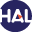# Volume 11 (2014)

Latest addition : 1 September 2014.

• ### Formulations of two phase liquid gas compositional Darcy flows with phase transitions

1 September 2014,

Abstract

In this article, three formulations of two phase compositional Darcy flows taking into account phase transitions are compared. The first formulation is the so called natural variable formulation commonly used in reservoir simulation, the second has been introduced in  and uses the phase pressures, saturations and component fugacities as main unknowns, and the third is an extension to general compositional two phase flows of the pressure pressure formulation introduced in  in the case of two compo- nents. The three formulations are shown to lead to equivalent definitions of the phase transitions for our gas liquid thermodynamical model. Then, they are compared numerically in terms of solution and convergence of the Newton type non linear solver on several 1D and 3D test cases including gas appearance and liquid disappearance. The 3D discretization is based on the Vertex Approximate Gradient (VAG) scheme  and takes into account discontinuous capillary pressures.

Key words : Darcy flow, two-phase flow, phase transitions, composi- tional models, finite volume scheme, discontinuous capillary pressures

Paper presented by: Professor Thierry GallouetVoir sur HAL : https://hal.archives-ouvertes.fr/hal-00910366

• ### Application of an homogeneous model to simulate the heating of two-phase flows

21 May 2014,

Abstract This paper is dedicated to the simulation of two-phase flows on the basis of an homogeneous model that allows to account for the disequilibrium of the pressure, temperature and chemical potential (mass transfer). The numerical simulations are performed using a fractional step method treat- ing separately the convective part of the model and the source terms. On the basis of analytical solutions for the convective part of the model, nu- merical investigations are performed to compare different finite volume schemes. Eventually, a test case of the heating of a mixture of steam and water is presented.

Key words : Homogeneous model, two-phase flows, mass transfer.

Paper presented by: Professor Thierry GallouetVoir sur HAL : https://hal.archives-ouvertes.fr/hal-01114808

• ### An entropy preserving MOOD scheme for the Euler equations

28 January 2014,

Abstract The present work concerns the derivation of entropy stability properties to be satisfied by high-order accurate finite volume methods. Such a sta- bility turns out to be crucial when approximating the weak solutions of hyperbolic systems of conservation laws. In fact, several recent works pro- pose some kind of discrete entropy inequalities associated to high-order schemes. However, these entropy preserving schemes do not seem relevant to impose that the converged solution (in the sense of the Lax-Wendroff Theorem) satisfies the required entropy inequalities. We illustrate such a failure by exhibiting numerical schemes that, from one hand, satisfy en- tropy stability and, from the other hand, do not prevent numerical blow- up. Here, we recall the expected high-order discrete entropy inequalities to be certain that the approximate solution converges to an entropy solution. Equipped with these sufficient numerical entropy stability, we propose to extend the recently introduced high-order MOOD scheme to satisfy the required high-order entropy inequalities. In fact, the MOOD approach is based on an a posteriori estimation and it seems impossible to impose a posteriori the whole set of discrete entropy inequalities. We solve this dif- ficulty by considering a finite volume scheme, which involves (at least one) discrete entropy inequalities with a numerical transport property. From one selected numerical transport discrete entropy inequality, we establish that all the needed discrete entropy inequalities are satisfied. Arguing this specific numerical transport entropy, we derive the expected a posteriori entropy condition to get an entropy preserving high-order MOOD scheme. Numerical experiments illustrate the relevance of the suggested numerical procedure.

Keywords : Euler equations, numerical approximation, finite volume methods, high-order approximation, discrete entropy inequalities.

Paper presented by: Professor Thierry GallouetVoir sur HAL : https://hal.archives-ouvertes.fr/hal-01115334